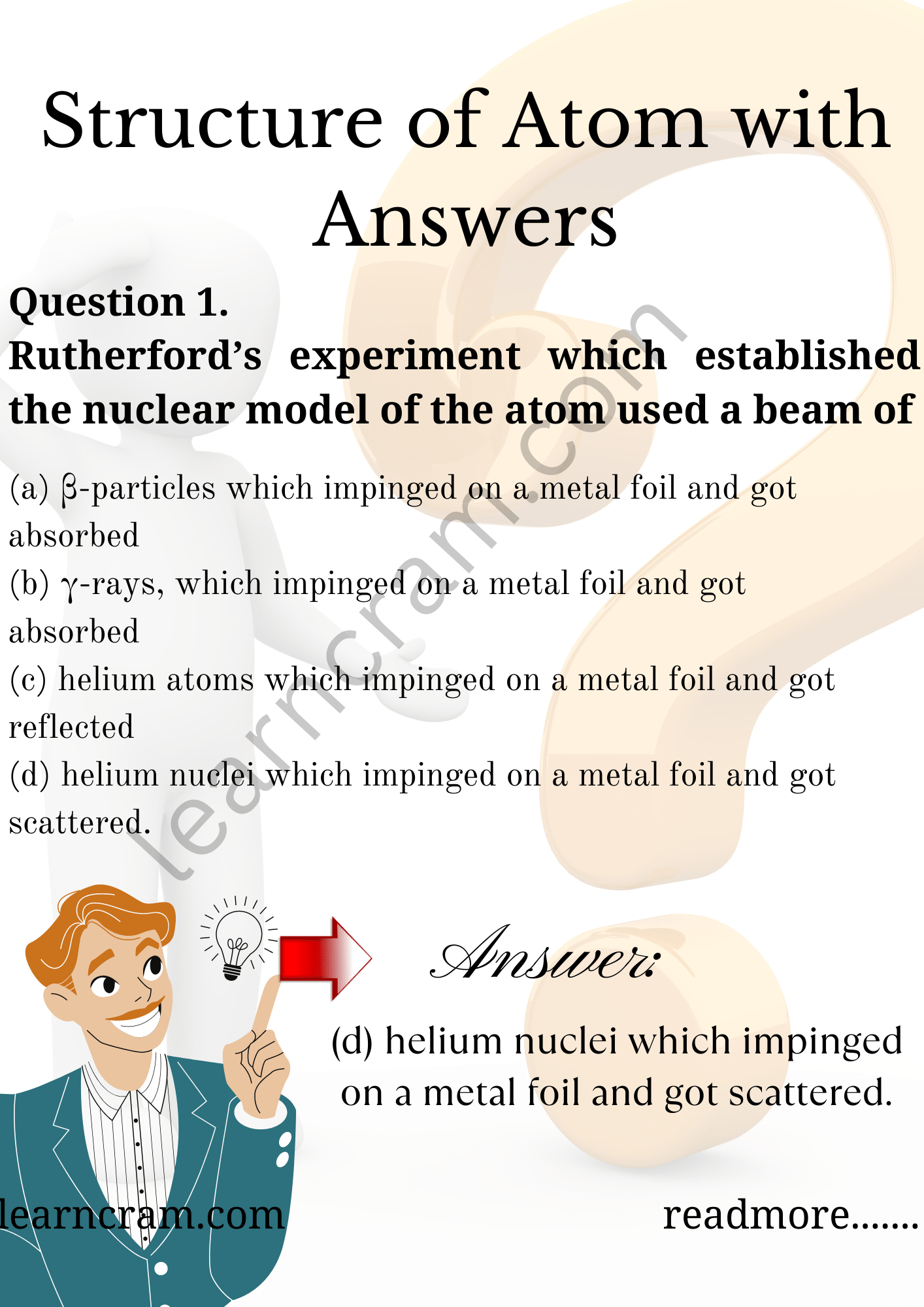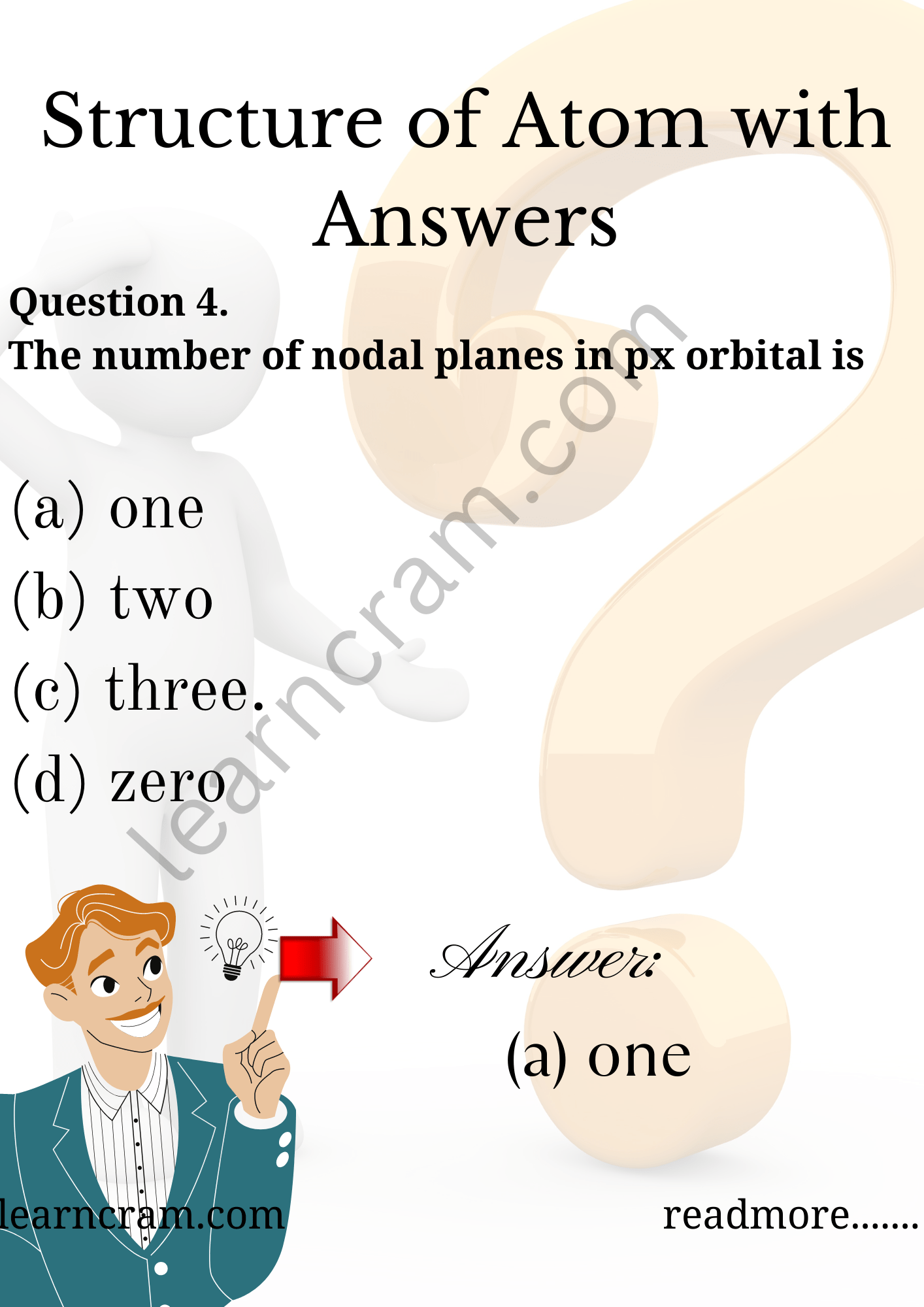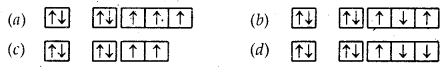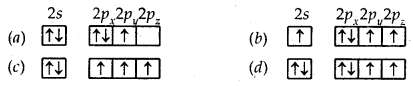# MCQ Questions for Class 11 Chemistry Chapter 2 Structure of Atom with Answers

We have compiled the NCERT MCQ Questions for Class 11 Chemistry Chapter 2 Structure of Atom with Answers Pdf free download covering the entire syllabus. Practice MCQ Questions for Class 11 Chemistry with Answers on a daily basis and score well in exams. Refer to the Structure of Atom Class 11 MCQs Questions with Answers here along with a detailed explanation.

## Structure of Atom Class 11 MCQs Questions with Answers

Multiple Choice Type Questions

Question 1.
Rutherford’s experiment which established the nuclear model of the atom used a beam of
(a) β-particles which impinged on a metal foil and got absorbed
(b) γ-rays, which impinged on a metal foil and got absorbed
(c) helium atoms which impinged on a metal foil and got reflected
(d) helium nuclei which impinged on a metal foil and got scattered.

Answer: (d) helium nuclei which impinged on a metal foil and got scattered.Question 2.
How many moles of electrons weigh one kilogram?
(a) 6.022 × 1023
(b) $$\frac {1}{9.108}$$ × 1031
(c) $$\frac {6022}{9.108}$$ × 1054
(d) $$\frac {1}{9.108 ×6.022}$$ × 108

Answer: (d) $$\frac {1}{9.108 ×6.022}$$ × 108

Question 3.
The wavelength associated #vith a golf ball weighing 200 g and moving at a speed of 5 m/h is of the order
(a) 10-10 m
(b) 10-20 m
(c) 10-30 m
(d) 10-40 m

Question 4.
The number of nodal planes in px orbital is
(a) one
(b) two
(c) three.
(d) zeroQuestion 5.
The electronic configuration of an element is 1s² 2s² 2p6 3s² 3p6 3d5 4s1. It represents
(a) excited state
(b) cationic states
(c) ground state
(d) anionic state

Question 6.
Azimuthal quantum number determines the
(a) size
(b) spin
(c) orientation
(d) angular momentum of orbitals

Answer: (d) angular momentum of orbitals

Question 7.
The electronic configuration of Cu2+ ion is
(a) [Ar] 4s1 3d8
(b) [Ar] 4s² 3d10 4p1
(c) [Ar] 4s1 3d10
(d) [Ar] 3d9

Question 8.
The total number of orbitals in a shell having principal quantum n is
(a) 2n
(b) n²
(c) 2n²
(d) (n + 1)

Question 9.
The ion iso-electronic with CO is
(a) O2
(b) N+2
(c) CN
(d) O+2

Question 10.
The line spectrum of hydrogen obtained in the visible region of light corresponds to
(a) Lyman series
(b) Balmer series
(c) Paschen series
(d) Brackett series

Question 11.
If the nitrogen atom had electronic configuration 1s², it would have energy lower than that of the normal ground state configuration 1s² 2s² 2p³, because the electrons would be closer to the nucleus. Yet, 1s² is not observed because it isolates.
(a) Heisenberg’s Uncertainty Principle
(b) Hund’s rule
(c) Pauli Exclusion Principle
(d) Bohr postulate of stationary orbits

Question 12.
The quantum numbers + 1/2 and – 1/2 for the electron spin represent
(a) rotation of the electron in clockwise and anticlockwise direction respectively
(b) rotation of the electron in anticlockwise and clockwise direction respectively
(c) magnetic moment of the electron pointing up and down respectively
(d) two quantum mechanical spin states which have no classified analogue

Answer: (d) two quantum mechanical spin states which have no classified analogue

Question 13.
The energy of electron in the first Bohr orbit of H atom is 13.6 eV. The possible energy value (s) of the excited state(s) for electron in Bohr orbitals of hydrogen is (are)
(a) – 3.4 eV
(b) -4.2eV
(c) – 6.8 eV
(d) + 6.8 eV

Question 14.
The last entering electron in an element has quantum number n = 3, l = 2, m = + 2 and s = + 1 /2. The atomic number of the element will be
(a) 13
(b) 21
(c) 29
(d) 39

Question 15.
What is the wavelength of light. Given energy = 2.91 × 10-19], h = 6.36 × 10-34 Js, c = 3.0 × 108 m/s?
(a) 6.56 nm
(b) 656 nm
(c) 0.656 nm
(d) 65.6 nm

Question 16.
Bohr atomic model can explain
(a) the spectrum of hydrogen atom only
(b) spectrum of an atom or ion containing one electron only
(c) the spectrum of hydrogen molecule
(d) the solar spectrum

Answer: (b) spectrum of an atom or ion containing one electron only

Question 17.
Magnetic quantum number specifies
(a) orbital size
(b) orbital shape
(c) orbital orientation
(d) nuclear stability

Question 18.
Which of the following set of quantum numbers belong to highest energy?
(a) n = 4, l = 0, m = 0, s = + $$\frac {1}{2}$$
(b) n = 3, l = 0, m = 0, s = + $$\frac {1}{2}$$
(c) n = 2, l = 1, m = 1, s = + $$\frac {1}{2}$$
(d) n = 3, l = 2, m = 1, s = + $$\frac {1}{2}$$

Answer: (d) n = 3, l = 2, m = 1, s = + $$\frac {1}{2}$$

Question 19.
Ground state electronic configuration of nitrogen is represented asQuestion 20.
The orbital diagram in which Aufbau principle is violated isFill in the Blanks

Question 1.
Wave functions of electrons in atoms and molecules are called ……………….

Question 2.
The 2px, 2px 2z orbitals of atoms have identical shapes but differ in their ………………

Question 3.
Cathode rays produce ……………… when they strike against the surface of a hard metal like Tungstun.

Question 4.
Cathode rays produce ……………….. on striking ZnS screen.

Question 5.
For n = 3, the values of l are ………………..

Question 6.
The probability of finding the electron at any point around the nucleus is found from the values of ……………….

Question 7.
The values of n, l and m for the last electron of atom with electronic configuration 2, 8, 3 are ………………..

Answer: 3, 1, -1 (or + 1 or 0)

Question 8.
The outermost electronic configuration of Cr is ……………….

Question 9.
The light radiations with discrete quantities of energy are called …………………

Question 10.
$$\frac {h}{λ}$$ is the angular momentum of the electron in the ………………. orbit of He+.

True or False Statements

Question 1.
The nucleus of an atom contains electrons and protons.

Question 2.
2H is more reactive than 1H.

Question 3.
The energy of the electron in 3d orbital is less than that in 4s orbital.

Question 4.
F has more electrons than Na+.

Question 5.
Three electrons in p-orbital remains unpaired.

Question 6.
For a given value of l, the shape of orbital is independent of the value of n.

Question 7.
Cu+ is less stable than Cu2+.

Question 8.
2nd orbital is larger in size than Is orbital.

Question 9.
The energy of an electron in 3d orbital is less than that in the 4s orbital in the hydrogen atom.

Question 10.
Angular momentum of electron in a shell is quantized, was derived by Bohr.

Question 11.
For s-orbitals, orbital angular momentum is zero.

Question 12.
The angular momentum of a moving electron is an integral multiple of h/2π.

Question 13.
Bohr’s atom consists of circular orbits.

Question 14.
Two electrons can have the same values for n, l and m but should have different values of s.

Question 15.
The maximum number of electrons which can be accommodated in an orbital is 2n².